# Self-Indexed Arrays

(Redirected from Self-Indexed Table)

Each dimension of an array is identified by an Index. Usually, each index is defined as an index object, which appears in a Diagram like this:But, it's also possible for a standard variable to contain an index value in addition to its actual value. This index value is called a "Self index" because the actual array value of the variable is indexed by the variable's own index value. An array value of another variable may also use the self index of this variable. Self-indexes can arise and be useful in several situations.

## Create a self-indexed variable

Here's one way to create a self-indexed variable:

First, drag a variable node to the diagram and name it X. Press thebutton on the toolbar to edit the definition and select "Table" from the definition type dropdown: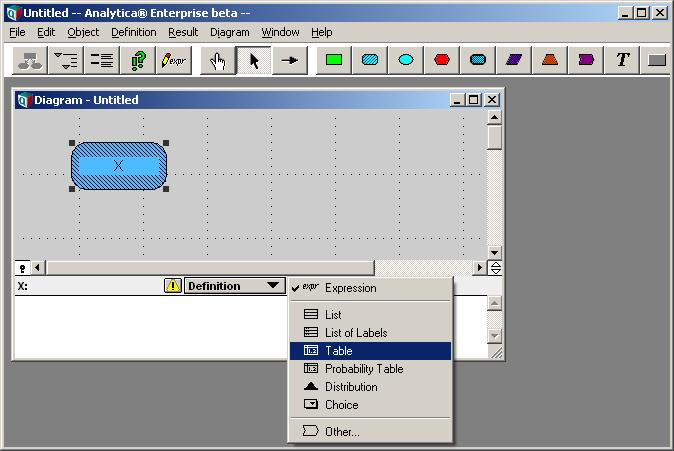In the index chooser dialog, select the "X (Self)" index and press OK.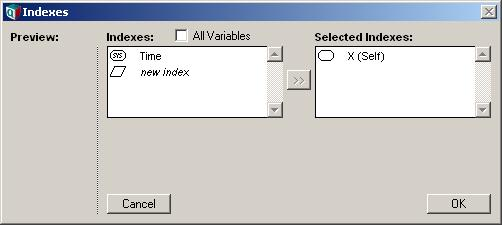You have now just created an edit table with a self index.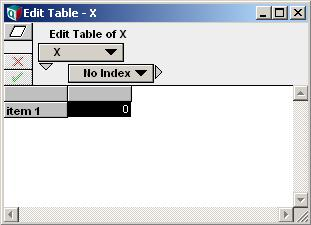Notice that the row-index is X, and the edit table itself is for X. Here the row headings are the index values for X, the table body cells hold the main value for X. Next, fill in both the self-index value and the body cells with values, as follows.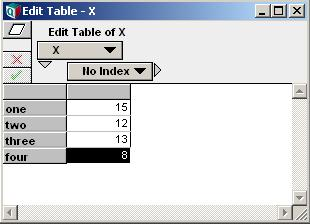As we see here, the variable's self-index value is usually different from its actual value.

## Expression Context

When a variable has both a main value and an index value, what happens when its identifier is used in an expression? (Side note: In general, a variable may have a Mid value and a probabilistic, or sample, value. See Evaluation Modes for more detail. Here we refer to either of these as the main value.)

As it turns out, Analytica can determine whether to use the main value or the index value based on the context in which the identifier appears. A simple example is this:

Sum(X, X)

This expressions sums the value of «X» along the «X» index. So, when «X» is a self-indexed variable, the main value is used for the first parameter, and its self-index value is used for the second parameter.

In general, the parameters of a function (whether built-in or user-defined) are declared using various Function Parameter Qualifiers. When a parameter is declared using the IndexType qualifier, then identifiers passed for that parameter are interpreted as indexes. Parameters that are declare otherwise are interpreted as values. (Note: There are further subtleties involved when a parameter is declared as a VariableType, which are beyond the scope of this introduction).

## The IndexType qualifier

When you define your own user-defined function (UDF), you might declare a parameter to be an index. This creates a local variable that is an alias for the index that is passed in. If this local variable is used in a value context, the index value (and not the main value) is used. So, for example, consider the following function:Suppose, using the self-indexed array defined earlier, we call this function using JoinIndex():

JoinIndex(X)

The result obtained is:

"one, two, three, four"

Compare this to what we would get by evaluating Join() in Join( X, X,' ,'), which results in "15,12,13,8".

Three special functions exist to explicit control which value to use from within an expression. These are applicable to expressions being evaluated in a value context. These are: Mid, Sample and IndexValue. The Mid and Sample functions provide access to the main values of the variable (see Evaluation Modes for the distinction), while the IndexValue function returns the index value. Using the IndexValue function, we could for example join the index value of X using

Join(IndexValue(X), X, ",")

yielding

"one, two, three, four"

## Copy Index

Using the X variable defined above, suppose we wish to define an index, J, having the same values that X has. We now have two choices - should this new index be composed of X's index values, i.e., ['one', 'two', 'three', 'four'], or of X's result values, i.e., [15,12,13, 8]? Either is possible:

CopyIndex(X) → [15, 12, 13, 8]
CopyIndex( IndexValue(X) ) → ['one', 'two', 'three', 'four']

Note: There are several alternative ways of copying the index value. The IndexValue function is new to Analytica 4.0, but for earlier versions one could use

Subset(Array(X,1)) → ['one', 'two', 'three', 'four']

## List Definitions

A second way that index values often arise in Analytica is by defining a variable as a list of identifiers, or as a list of expressions. This is often very convenient when creating a report, bringing several results together in a single table view.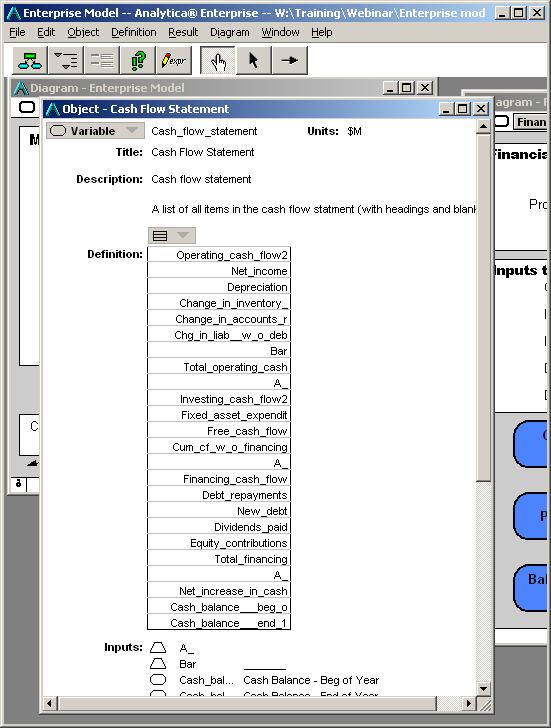The variable shown in the preceding screenshot is defined as a list of identifiers. When this variable is evaluated, it has both a self-index (the list of identifiers) and a value, obtained by evaluating each of the variables appearing in the table and joining them into a single array.

In this model, most of the variables appearing in the list evaluate to a result that is indexed by Time. Therefore, the value obtained is two-dimensional, indexed by itself (Cash_flow_statement) and by Time.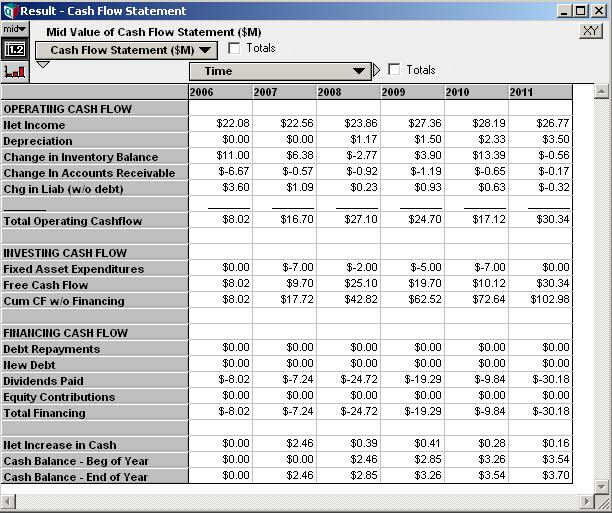Side note: When Analytica displays a self-indexed result, and the self-index is composed of object identifiers, then Analytica uses separate number formats for each row (or column if pivoted), taking the number format for the corresponding row from the identifier that appears in the self-index. This provides a capability to format each row of a table differently.

The value of IndexValue(Cash_flow_statement) in this example is composed of a list of varTerms, essentially handles to Analytica objects. In advanced Analytica modeling, these varTerms can be utilized for meta-level inference and button scripting (performing inference about the model itself). Meta-level inference is beyond the scope of the current article, but we point this out for readers who may already be at this level of expertise.

## Self-Indexes from the Domain

When the domain attribute contains an explicit list, either a list of expressions, list of labels, or index-domain, Analytica treats the domain value as the variable's self-index. This is often used when defining a Choice definition, DetermTable or ProbTable definition. For example, in a ProbTable, the main value (usually the Sample) is the randomly-sampled set of values, but the IndexValue for the variable is the set of all possible values.

The domain values can also be accessed using:

domain of X

It should be noticed that the treatment of the domain value as a self-index value is a functionality that Lumina plans to eventually eliminate. If you wish to refer to the domain value of a variable, it is preferable to use "domain of X" rather than IndexValue(X) to ensure forward compatibility with future releases of Analytica.

The use of the domain in a Choice definition, or to index a DetermTable or ProbTable will continue to be valid in future releases. However, when the changes we envision are eventually enacted, we could allow for the possibility of a multi-select Choice control, for example. In such a case, the main and index values could be a subset of the domain value.

Note: Few models utilize the domain of a variable as a self-index outside their use of Choice(Self, n), DetermTable or ProbTable, so this change only impacts models that do fairly unusual things.